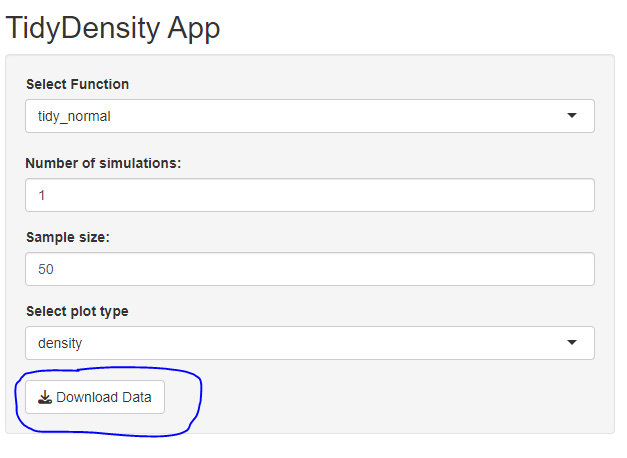# Exploring Distributions with {shiny} and {TidyDensity} Part 3

rtip
shiny
tidydensity
Author

Steven P. Sanderson II, MPH

Published

April 20, 2023

# Introduction

In the previous post we allowed users to choose a distribution and a plot type. Now, we want to allow users to download a .csv file of the data that is generated.

In the UI, we added a `downloadButton` with `outputId = "download_data"` and `label = "Download Data"`. In the server, we added a `downloadHandler` that takes a `filename` and content function. The `filename` function returns the name of the file to be downloaded (in this case, we used the selected function name as the file name with “.csv” extension). The content function writes the reactive data to a CSV file using the write.csv function. The `downloadHandler` returns the file to be downloaded when the button is clicked.

See here:# UI Section

Here is the update to the UI Section

``````# Define UI
ui <- fluidPage(
titlePanel("TidyDensity App"),
sidebarLayout(
sidebarPanel(
selectInput(inputId = "functions",
label = "Select Function",
choices = c(
"tidy_normal",
"tidy_bernoulli",
"tidy_beta",
"tidy_gamma"
)
),
numericInput(inputId = "num_sims",
label = "Number of simulations:",
value = 1,
min = 1,
max = 15),
numericInput(inputId = "n",
label = "Sample size:",
value = 50,
min = 30,
max = 200),
selectInput(inputId = "plot_type",
label = "Select plot type",
choices = c(
"density",
"quantile",
"probability",
"qq",
"mcmc"
)
),
),
mainPanel(
plotOutput("density_plot"),
DT::dataTableOutput("data_table")
)
)
)``````

# Server Section

Here is the update to the Server section.

``````# Define server
server <- function(input, output) {

# Create reactive data
data <- reactive({
# Call selected function with user input
match.fun(input\$functions)(.num_sims = input\$num_sims, .n = input\$n)
})

# Create density plot
output\$density_plot <- renderPlot({
# Call autoplot on reactive data
p <- data() |>
tidy_autoplot(.plot_type = input\$plot_type)

print(p)
})

# Create data table
output\$data_table <- DT::renderDataTable({
# Return reactive data as a data table
DT::datatable(data())
})

filename = function() {
paste0(input\$functions, ".csv")
},
content = function(file) {
write.csv(data(), file, row.names = FALSE)
}
)

}

# Run the app
shinyApp(ui = ui, server = server)``````

# Conclusion

With these changes, the user can now export the data to a .csv file by clicking the “Export Data” button and selecting where to save the file.

I hope this update to the TidyDensity app will make it more useful for your data analysis needs. If you have any questions or feedback, please feel free to let me know, and as usual…Steal this Code!! Modify for yourself and see what you come up with.

Here is the entire script:

``````library(shiny)
library(TidyDensity)
library(tidyverse)
library(DT)

# Define UI
ui <- fluidPage(
titlePanel("TidyDensity App"),
sidebarLayout(
sidebarPanel(
selectInput(inputId = "functions",
label = "Select Function",
choices = c(
"tidy_normal",
"tidy_bernoulli",
"tidy_beta",
"tidy_gamma"
)
),
numericInput(inputId = "num_sims",
label = "Number of simulations:",
value = 1,
min = 1,
max = 15),
numericInput(inputId = "n",
label = "Sample size:",
value = 50,
min = 30,
max = 200),
selectInput(inputId = "plot_type",
label = "Select plot type",
choices = c(
"density",
"quantile",
"probability",
"qq",
"mcmc"
)
),
),
mainPanel(
plotOutput("density_plot"),
DT::dataTableOutput("data_table")
)
)
)

# Define server
server <- function(input, output) {

# Create reactive data
data <- reactive({
# Call selected function with user input
match.fun(input\$functions)(.num_sims = input\$num_sims, .n = input\$n)
})

# Create density plot
output\$density_plot <- renderPlot({
# Call autoplot on reactive data
p <- data() |>
tidy_autoplot(.plot_type = input\$plot_type)

print(p)
})

# Create data table
output\$data_table <- DT::renderDataTable({
# Return reactive data as a data table
DT::datatable(data())
})

filename = function() {
paste0(input\$functions, ".csv")
},
content = function(file) {
write.csv(data(), file, row.names = FALSE)
}
)

}

# Run the app
shinyApp(ui = ui, server = server)``````

Voila!